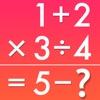# FX Math Junior Problem Solver - Lumos Educational App Store31
Price - Free
\$0

#### DESCRIPTION:

Will guide you how to solve your math homework and textbook problems, anytime, anywhere.FX Math Junior is a comprehensive math software, based on an automatic mathematical problem solving engine, including: - Over 400 sample arithmetic problems and fully animated solution steps - Automatic problem solving and generation of fully animated step-by-step procedures for problems typed in by users - User friendly math problem expression editor (WYSIWYG mode) FX Math Junior covers problems at the level of Grade 3-7 Math and Pre-Algebra courses: - Number evaluations - Add, subtract, multiply, divide -

#### OVERVIEW:

FX Math Junior Problem Solver is a free educational mobile app By Euclidus Inc.It helps students in grades 3,4,5,6,7 practice the following standards 3.OA.A.3.

This page not only allows students and teachers download FX Math Junior Problem Solver but also find engaging Sample Questions, Videos, Pins, Worksheets, Books related to the following topics.

1. 3.OA.A.3 : Use multiplication and division within 100 to solve word problems in situations involving equal groups, arrays, and measurement quantities, e.g., by using drawings and equations with a symbol for the unknown number to represent the problem. .

3
4
5
6
7

#### STANDARDS:

3.OA.A.3

Developer: Euclidus Inc

Software Version: 2.2

Category: Education

Release Date: 2014-04-24T09:53:58Z### RELATED APPSEdSearch WebSearch